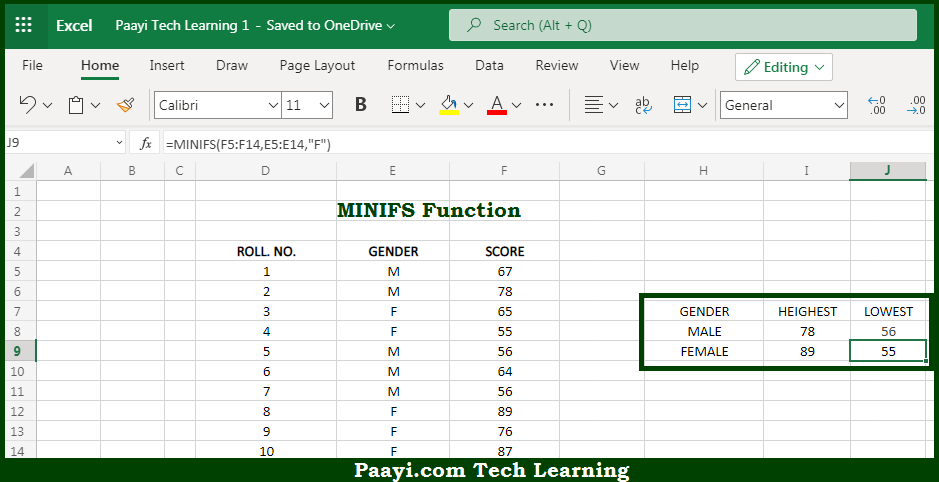# Learn How to Use Microsoft Excel MINIFS Function

Written by | 0 Comments | 380 Views

In this article, you will learn how to use the Microsoft Excel MINIFS function and its prime function in Microsoft Excel. You will also get to know the Microsoft Excel MINIFS function return value and syntax with the help of some examples.

Microsoft Excel MINIFS Function

The main purpose of the Microsoft Excel MINIFS function is to return the minimum value from the given array with criteria. That implies, with the help of MINIFS function you can able to return the smallest numeric value in a range of values that meets one or more criteria. It should be noted that MINIFS can be used with criteria based on dates, numbers, text, and other conditions. MINIFS supports logical operators (>,<,<>,=) and wildcards (*,?) for partial matching. So, with the help of MINIFS function, you can able to get the smallest value from the range of values provided with the criteria.

Return Value of MINIFS Function

The return value will be the minimum value.

Syntax of MINIFS Function

=MINIFS(min-range, range1, criteria1, [range2], [criteria2], ...)

Where the arguments:

• min-range: This is the range or value used to determine the maximum.
• range1: This is the first range to evaluate.
• criteria1: This is the criteria to use on range1.
• range2: This is the second range to evaluate.
• criteria2: This is the criteria to use on range2.

## How to Use Microsoft Excel MINIFS Function?So we know that Microsoft Excel MINIFS function you can able to return the minimum value from the given array with criteria. That implies, with the help of MINIFS function you can able to return the smallest numeric value in a range of values that meets one or more criteria. It should be noted that MINIFS can be used with criteria based on dates, numbers, text, and other conditions. MINIFS supports logical operators (>,<,<>,=) and wildcards (*,?) for partial matching. So, with the help of MINIFS function, you can able to get the smallest value from the range of values provided with the criteria.